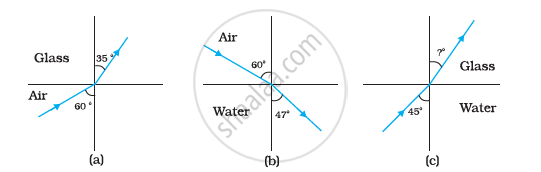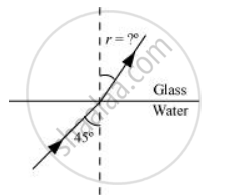# Figures (A) and (B) Show Refraction of a Ray in Air Incident at 60° with the Normal to a Glass-air and Water-air Interface, Respectively. - Physics

Numerical

Figures (a) and (b) show the refraction of a ray in air incident at 60° with the normal to a glass-air and water-air interface, respectively. Predict the angle of refraction in the glass when the angle of incidence in water is 45° with the normal to a water-glass interface [Figure (c)].#### Solution

As per the given figure, for the glass-air interface:

Angle of incidence, i = 60°

Angle of refraction, r = 35°

The relative refractive index of glass with respect to air is given by Snell’s law as:

μ_"g"^"a" = (sin "i")/(sin "r")

= (sin 60°)/(sin 35°)

= (0.8660)/(0.5736)

= 1.51 ......(1)

As per the given figure, for the air-water interface:

Angle of incidence, i = 60°

Angle of refraction, r = 47°

The relative refractive index of water with respect to air is given by Snell’s law as:

μ_"w"^"a" = (sin "i")/(sin "r")

= sin 60/sin 47

= (0.8660)/(0.7314)

= 1.184 ....(2)

Using (1) and (2), the relative refractive index of glass with respect to water can be obtained as:

μ_"g"^"w" = (μ_"g"^"a")/(μ_"w"^"a")

= 1.51/1.184

= 1.275

The following figure shows the situation involving the glass-water interface.Angle of incidence, i = 45°

Angle of refraction = r

From Snell’s law, r can be calculated as:

(sin "i")/(sin "r") = μ_"g"^"w"

(sin 45°)/sin "r" = 1.275

sin "r" = (1/(sqrt2))/1.275 = 0.5546

∴ r = sin^(-1) (0.5546) = 38.68°

Hence, the angle of refraction at the water-glass interface is 38.68°.

Concept: Refraction
Is there an error in this question or solution?

#### APPEARS IN

NCERT Physics Part 1 and 2 Class 12
Chapter 9 Ray Optics and Optical Instruments
Exercise | Q 9.4 | Page 344
NCERT Class 12 Physics Textbook
Chapter 9 Ray Optics and Optical Instruments
Exercise | Q 4 | Page 345

Share